Test: Series- 2

# Test: Series- 2 - Class 10

Test Description

## 10 Questions MCQ Test Olympiad Preparation for Class 10 - Test: Series- 2

Test: Series- 2 for Class 10 2023 is part of Olympiad Preparation for Class 10 preparation. The Test: Series- 2 questions and answers have been prepared according to the Class 10 exam syllabus.The Test: Series- 2 MCQs are made for Class 10 2023 Exam. Find important definitions, questions, notes, meanings, examples, exercises, MCQs and online tests for Test: Series- 2 below.
Solutions of Test: Series- 2 questions in English are available as part of our Olympiad Preparation for Class 10 for Class 10 & Test: Series- 2 solutions in Hindi for Olympiad Preparation for Class 10 course. Download more important topics, notes, lectures and mock test series for Class 10 Exam by signing up for free. Attempt Test: Series- 2 | 10 questions in 20 minutes | Mock test for Class 10 preparation | Free important questions MCQ to study Olympiad Preparation for Class 10 for Class 10 Exam | Download free PDF with solutions
 1 Crore+ students have signed up on EduRev. Have you?
Test: Series- 2 - Question 1

### Each of the following questions consists of five figures marked 1, 2, 3, 4 and 5. These figures form a series. Find out the one from the answer figures that will continue the series. Problem Figures: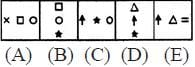Answer Figures: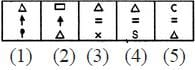Detailed Solution for Test: Series- 2 - Question 1

In each step, the first element moves to the third position and gets replaced by a new element; the second and the third elements move to the first and the second positions respectively and the entire figure rotates by 90°.

Test: Series- 2 - Question 2

### Each of the following questions consists of five figures marked 1, 2, 3, 4 and 5. These figures form a series. Find out the one from the answer figures that will continue the series. Problem Figures: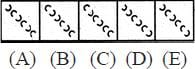Answer Figures: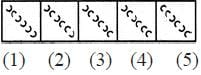Detailed Solution for Test: Series- 2 - Question 2

We can label the arcs as shown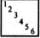. The arcs get inverted in the sequence (1 & 2), (3, 4 & 5), (6 & 1), (2, 3 & 4), (5 & 6).

Test: Series- 2 - Question 3

### Each of the following questions consists of five figures marked 1, 2, 3, 4 and 5. These figures form a series. Find out the one from the answer figures that will continue the series. Problem Figures: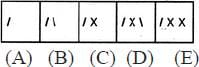Answer Figures: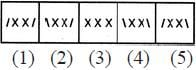Detailed Solution for Test: Series- 2 - Question 3

One extra line is added in each step in a set order.

Test: Series- 2 - Question 4

Each of the following questions consist of five figures marked 1, 2, 3, 4 and 5 called the Problem Figures followed by five other figures marked A, B, C, D and E called the Answer Figures. Select a figure from amongst the Answer Figures which will continue the same series as established by the five Problem Figures.

Problem Figures: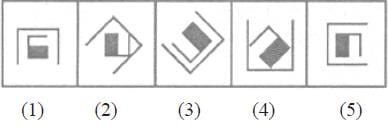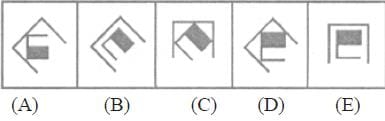Test: Series- 2 - Question 5

Each of the following questions consist of five figures marked 1, 2, 3, 4 and 5 called the Problem Figures followed by five other figures marked A, B, C, D and E called the Answer Figures. Select a figure from amongst the Answer Figures which will continue the same series as established by the five Problem Figures.

Problem Figures: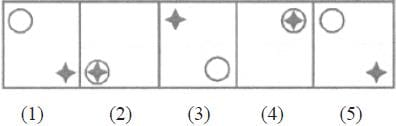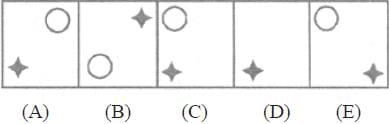Test: Series- 2 - Question 6

Each of the following problems, contains four Problem Figures marked 1, 2, 3, and 4 and five Answer Figures marked A, B, C, D and E. Select a figure from amongst the Answer figures which will continue the same series as given in the Problem Figures.

Problem Figures: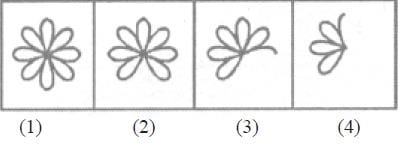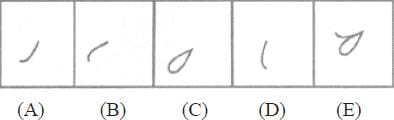Test: Series- 2 - Question 7

Each of the following problems, contains four Problem Figures marked 1, 2, 3, and 4 and five Answer Figures marked A, B, C, D and E. Select a figure from amongst the Answer figures which will continue the same series as given in the Problem Figures.

Problem Figures: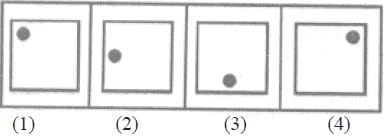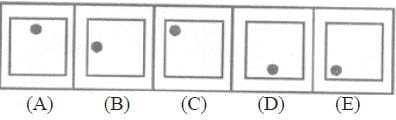Test: Series- 2 - Question 8

Each of the following problems, contains four Problem Figures marked 1, 2, 3, and 4 and five Answer Figures marked A, B, C, D and E. Select a figure from amongst the Answer figures which will continue the same series as given in the Problem Figures.

Problem Figures: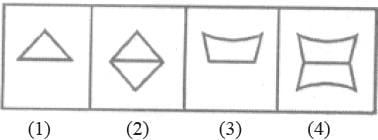Test: Series- 2 - Question 9

In each of the following questions, select a figure from amongst the four alternatives, which when placed in the blank space of figure (X) would complete the pattern.

Identify the figure that completes the pattern.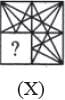Detailed Solution for Test: Series- 2 - Question 9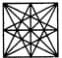Test: Series- 2 - Question 10

In each of the following questions, select a figure from amongst the four alternatives, which when placed in the blank space of figure (X) would complete the pattern.

Identify the figure that completes the pattern.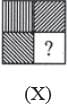Detailed Solution for Test: Series- 2 - Question 10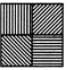## Olympiad Preparation for Class 10

11 videos|36 docs|201 tests
Information about Test: Series- 2 Page
In this test you can find the Exam questions for Test: Series- 2 solved & explained in the simplest way possible. Besides giving Questions and answers for Test: Series- 2, EduRev gives you an ample number of Online tests for practice

## Olympiad Preparation for Class 10

11 videos|36 docs|201 tests